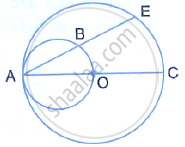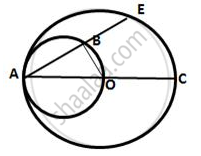Share

Books Shortlist

# In the Given Figure, Ac is a Diameter of a Circle, Whose Centre is O. a Circle is Described on Ao as Diameter. Ae, a Chord of the Larger Circle, Intersects the Smaller Circle at B. Prove that Ab = Be - ICSE Class 10 - Mathematics

ConceptChord Properties - the Perpendicular to a Chord from the Center Bisects the Chord (Without Proof)

#### Question

In the given figure, AC is a diameter of a circle, whose centre is O. A circle is described on AO
as diameter. AE, a chord of the larger circle, intersects the smaller circle at B. prove that AB =
BE.#### SolutionJoin OB,
Then ∠OBA = 90°
(Angle in a semicircle is a right angle)
i.e. OB⊥AE
We know the perpendicular drawn from the centre to a chord bisects the chord.
∴ AB = BE

Is there an error in this question or solution?

#### Video TutorialsVIEW ALL 

Solution In the Given Figure, Ac is a Diameter of a Circle, Whose Centre is O. a Circle is Described on Ao as Diameter. Ae, a Chord of the Larger Circle, Intersects the Smaller Circle at B. Prove that Ab = Be Concept: Chord Properties - the Perpendicular to a Chord from the Center Bisects the Chord (Without Proof).
S